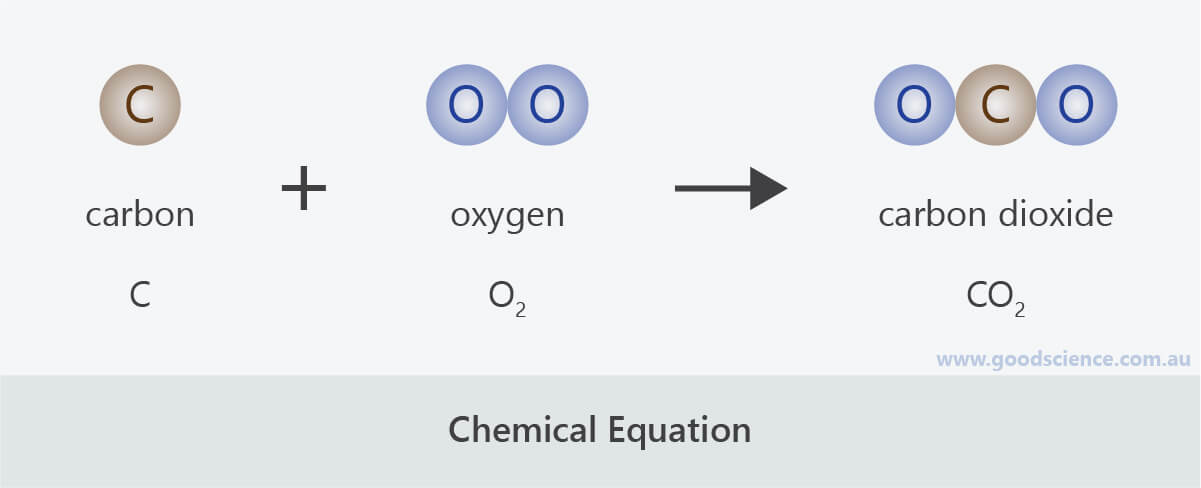Learning Objective

In this lesson we will learn how to represent chemical reactions as word equations and formula equations.

Learning Outcomes

By the end of this lesson you will be able to:

• Identify reactants and products in chemical reactions.
• Represent chemical reactions as word equations.
• Represent chemical reactions as formula equations.

Describing Chemical Reactions Using Equations

1 | Chemical Reactions

2 | Describing Chemical Reactions

3 | Chemical Equations

4 | Summary

Click images to preview the worksheet for this lesson and the Year 8 Chemistry Workbook (PDF and print versions)

## Chemical Reactions

• A chemical change can also be referred to as a chemical reaction.
• A chemical reaction involves one or more substances, known as reactants, interacting to form one or more new substances, known as products.
• Chemical reactions may involve one or more reactants.
• Similarly, they may involve one or more products.
• Example
• Consider the chemical reaction that takes place when magnesium ribbon is burned. The magnesium ribbon reacts with oxygen in the air to form magnesium oxide.
• In this reaction:
• ▸ There are two reactants – magnesium and oxygen.
• ▸ There is one product – magnesium oxide.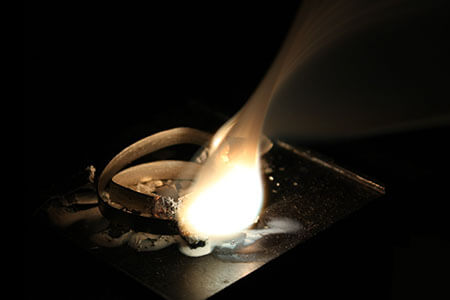When magnesium burns, it reacts with oxygen in the air to form magnesium oxide.

(Image: Capt. John Yossarian, Wikimedia Commons)

## Describing Chemical Reactions

• If we were to describe the chemical reaction that takes place when magnesium is burned, we might write something like:
• “When magnesium ribbon is burned over a Bunsen burner flame, a bright light is produced as it reacts with oxygen in the air, resulting in the formation of grey magnesium oxide powder.”
• Although describing chemical reactions in sentence form provides better descriptive detail about a reaction, it would be time consuming to do this each time we referred to a chemical reaction.
• Instead, chemical reactions are more commonly described in an abbreviated form, known as a chemical equation.
• Chemical equations have several advantages:
• They are shorter and simpler to write.
• They follow a consistent format, so that different people represent the same reaction in the same way.
• When using chemical symbols, they can be understood by people who speak different languages.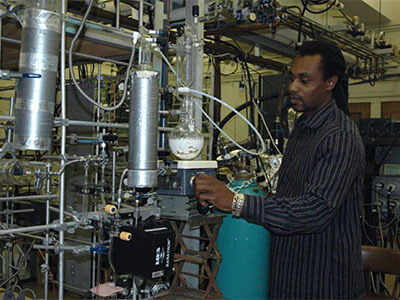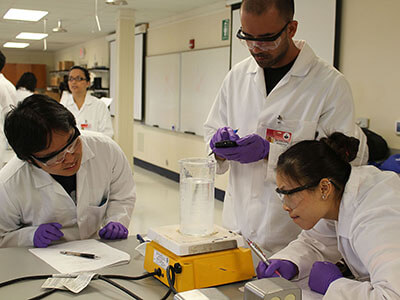Chemical equations can be understood by scientists all around the world.

(Images: National Cancer Institute, Wikimedia Commons; NASA/JPL; Kaiwikimom, Wikimedia Commons)

## Chemical Equations

• The general format of a chemical equation is:• Reactants are listed on the left and products are listed on the right.
• They are separated by an arrow, which shows that a chemical change has taken place. It is a short-hand way of saying “react to form”.
• * Many students, when learning to write chemical equations, use an equals sign instead of an arrow. But this implies that the reactants and products are the same thing, which is incorrect!
• If there is more than one reactant or product, they are separated by a plus sign, as shown below.• There are two main types of chemical equations – word equations and formula equations.
• We will discuss both of these next.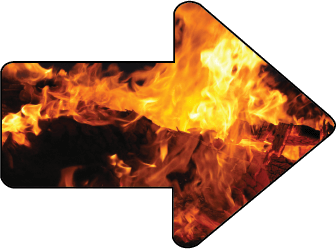The arrow in a chemical equation indicates that a chemical change has converted the reactants on the left into the products on the right.

(Image: Meditations, Pixabay)

### Word Equations

• Word equations contain the chemical names of all reactants and products.
• It is important that common names are not used. For example, if the word equation was to describe the rusting of iron, use ‘iron oxide’ rather than ‘rust’.
• Example 1
• The word equation for the burning of magnesium ribbon is:
magnesium  +  oxygen    magnesium oxide
• Example 2
• The word equation for the reaction between baking soda and vinegar is:
sodium hydrogen carbonate  +  acetic acid    sodium acetate  +  carbon dioxide  +  water

### Formula Equations

• Formula equations contain the chemical symbols of all reactants and products.
• Example 1
• The formula equation for the burning of magnesium ribbon is:
Mg  +  O2    MgO
• Example 2
• The formula equation for the reaction between baking soda and vinegar is:
NaHCO3  +  CH3COOH    NaCH3COO  +  CO2  +  H2O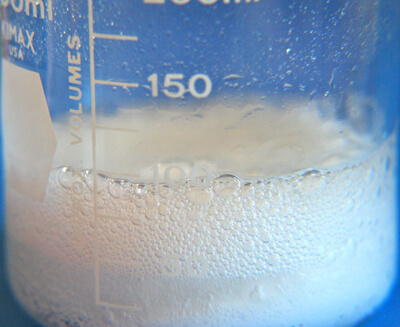The word equation describing the chemical reaction between baking soda and vinegar uses their scientific names: sodium hydrogen carbonate and acetic acid.
The formula equation describing the chemical reaction between baking soda and vinegar uses their chemical formulas: NaHCO3 and CH3COOH.

(Image: katerha, Wikimedia Commons)

## Summary

• A chemical change can also be referred to as a chemical reaction.
• A chemical reaction involves one or more substances, known as reactants, interacting to form one or more new substances, known as products.
• There are two main ways that chemical reactions can be described:
• In full sentences.
• As chemical equations.
• The general format of a chemical equation is:
•• The reactants and products are separated by an arrow, which shows that a chemical change has taken place.
• There are two types of chemical equations – word equations and formula equations.
• Word equations contain the chemical names of reactants and products.
• Formula equations contain the chemical symbols of reactants and products.